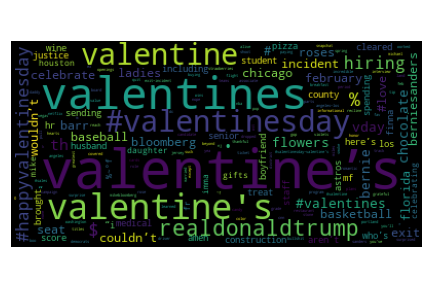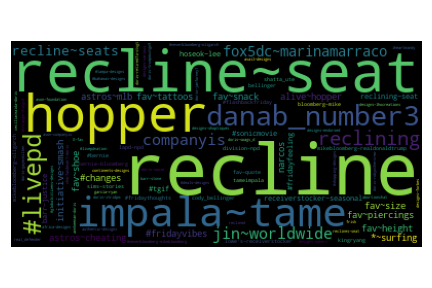# Vocabulary¶This module deals with the data of tokens and their frequency obtained from collected tweets per day. It can be used to replicate EvoMSA.base.EvoMSA(B4MSA=True) pre-trained model, to develop text models. and to analyze the tokens used in a period.

## Analyzing the vocabulary (i.e., tokens) on a day¶

Vocabulary class can also be used to analyze the tokens produced on a particular day or period. In the next example, let us examine the February 14th, 2020.

>>> from text_models import Vocabulary
>>> day = dict(year=2020, month=2, day=14)
>>> voc = Vocabulary(day, lang="En")
>>> voc.voc.most_common(3)
[('the', 691141), ('to', 539942), ('i', 518791)]


As can be seen, the result is not informative about the events that occurred in the day. Perhaps by removing common words would produce an acceptable representation.

>>> voc.remove(voc.common_words())
>>> voc.voc.most_common(3)
[('valentine’s', 22137), ("valentine's", 21024), ('valentines', 20632)]


## Word Clouds¶

The other studies that can be performed with the library are based on tokens and their frequency per day segmented by language and country. This information can serve to perform an exploratory data analysis; the following code creates a word cloud using words produced in the United States in English on February 14, 2020.

>>> from text_models import Vocabulary
>>> day = dict(year=2020, month=2, day=14)
>>> voc = Vocabulary(day, lang="En", country="US")


The tokens used to create the word cloud are obtained after removing the q-grams, the emojis, and frequent words.

>>> voc.remove_emojis()
>>> voc.remove(voc.common_words())


The word cloud is created using the library WordCloud. The first two lines import the word cloud library and the library to produce the plot. The third line then produces the word cloud, and the fourth produces the figure; the last is just an aesthetic instruction.

>>> from wordcloud import WordCloud
>>> from matplotlib import pylab as plt
>>> word_cloud = WordCloud().generate_from_frequencies(voc)
>>> plt.imshow(word_cloud)
>>> _ = plt.axis("off")As shown in the previous word cloud, the most frequent tokens are related to Valentines’ day. A procedure to retrieve other topics that occurred on this day is to remove the previous years’ frequent words.

>>> voc.remove(voc.day_words(), bigrams=False)


The word cloud is created using a similar procedure being the only difference the tokens given to the class.

>>> word_cloud = WordCloud().generate_from_frequencies(voc)
>>> plt.imshow(word_cloud)
>>> _ = plt.axis("off")## Similarity between Spanish dialects¶

The tokens and their frequency, grouped by country, can be used to model, for example, the similarity of a particular language in different countries. In particular, let us analyze the similarity between Spanish-speaking countries. The procedure followed is to take randomly 30 days, from January 1, 2019, to December 21, 2021 on Spanish-speaking countries.

>>> from text_models.utils import date_range
>>> import random
>>> NDAYS = 30
>>> init = dict(year=2019, month=1, day=1)
>>> end = dict(year=2021, month=12, day=31)
>>> dates = date_range(init, end)
>>> random.shuffle(dates)
>>> countries = ['MX', 'CO', 'ES', 'AR',
'PE', 'VE', 'CL', 'EC',
'GT', 'CU', 'BO', 'DO',
'HN', 'PY', 'SV', 'NI',
'CR', 'PA', 'UY']
>>> avail = Vocabulary.available_dates
>>> dates = avail(dates, n=NDAYS,
countries=countries,
lang="Es")


Once the dates are selected, it is time to retrieve the tokens from the Spanish-speaking countries.

>>> from text_models import Vocabulary
>>> vocs = [Vocabulary(dates.copy(), lang="Es", country=c)
for c in countries]


For all the countries, it is kept only the first $$n$$ tokens with higher frequency where $$n$$ corresponds to the 10% of the country’s vocabulary with less number of tokens.

>>> _min = min([len(x.voc) for x in vocs])
>>> _min = int(_min * .1)
>>> tokens = [x.voc.most_common(_min)
for x in vocs]
>>> tokens = [set(map(lambda x: x, i))
for i in tokens]


The Jaccard similarity matrix is defined in set operations. The first instruction of the following line transforms the vocabulary into a set. It can be seen that the output is a list of sets, each one corresponding to a different country. The second instruction builds the matrix. There are two nested loops, each one iterating for the country sets.

>>> X = [[len(p & t) / len( p | t)
for t in tokens] for p in tokens]


Each row of the Jaccard similarity matrix can be as the country signature, so in order to depict this signature in a plane, we decided to transform it using Principal Component Analysis. The following code transforms the matrix into a matrix with two columns.

>>> from sklearn.decomposition import PCA
>>> X = PCA(n_components=2).fit_transform(X)


Given that a two-dimensional vector represents each country, one can plot them in a plane using a scatter plot. The second line of the following code plots the vectors in a plane; meanwhile, the loop sets the country code close to the point.

>>> from matplotlib import pylab as plt
>>> plt.plot(X[:, 0], X[:, 1], "o")
>>> for l, x in zip(countries, X):
>>>   plt.annotate(l, x)## text_models.vocabulary¶

class text_models.vocabulary.BagOfWords(tokens: Union[str, List[str]] = 'Es')[source]

Bag of word model using TFIDF and text_models.vocabulary.Tokenize

Parameters

tokens ([str|List]) – Language (Ar|En|Es) or list of tokens

>>> from EvoMSA.tests.test_base import TWEETS
>>> from microtc.utils import tweet_iterator
>>> from text_models.vocabulary import BagOfWords
>>> tw = list(tweet_iterator(TWEETS))
>>> BoW = BagOfWords().fit(tw)
>>> BoW['hola mundo']
[(758, 0.7193757438600711), (887, 0.6946211479258095)]

fit(X: List[Union[str, dict]]) [source]

Train the Bag of words model

id2word(id: int) str[source]

Token associated with id

Parameters

id (int) – Identifier

property tokenize: Tokenize
transform(data: List[str]) csr_matrix[source]

Transform a list of text to a Bag of Words using TFIDF

class text_models.vocabulary.TextModel(docs=None, threshold=0, lang=None, negation=False, stemming=False, stopwords='none', **kwargs)[source]
text_transformations(text)[source]
>>> tm = TextModel(**TM_ARGS)
>>> tm.text_transformations('@user abd')
'~abd~'

class text_models.vocabulary.Tokenize(tm_args: Dict[str, Any] = {'del_dup': False, 'del_punc': True, 'emo_option': 'none', 'hashtag_option': 'none', 'num_option': 'none', 'url_option': 'delete', 'usr_option': 'delete'})[source]

Tokenize transforms a text into a sequence, where each number identifies a particular token; the q-grams that are not found in the text are ignored.

>>> from text_models import Tokenize
>>> tok = Tokenize().fit(["hi~mario", "mario"])
>>> tok.transform("good morning mario")


fit(tokens: List[str]) [source]

Train the tokenizer.

Parameters

tokens (List[str]) – Vocabulary as a list of tokens

id2word(id: int) str[source]

Token associated with id

Parameters

id (int) – Identifier

property textModel

Text model, i.e., :py:class::b4msa.text_model.TextModel

transform(texts: Union[Iterable[str], str]) List[Union[List[int], int]][source]

Transform the input into a sequence where each element represents a token in the vocabulary (i.e., text_models.vocabulary.Tokenize.vocabulary)

property vocabulary: Dict[str, int]

Vocabulary used

class text_models.vocabulary.TopicDetection(date, lang: str = 'En', country: str = 'US', window: int = 2)[source]

TopicDetection Class is used to visualize the topics of interest for a specified date based on the tweets from Twitter for that day

Parameters
• date – Date provided in format dict(year=yyyy, month=mm, day=dd)

• lang (str) – Language (Ar, En, or Es)

• country (str) – Two letter country code

static laplace_smoothing(voc1, voc2) {}[source]

Uses Laplace smoothing to handle words that appear in voc1 but not in voc2

static probability(voc) {}[source]

Calculate the probability of each word appearing in vocab

similar_date()[source]

Use cosine similarity to return the most similar date from around the same date in the previous year.

topic_wordcloud(figname: str = 'wordcloud')[source]

Uses WordCloud library to display the topic

Use Laplace Smoothing and compare the vocabs from the date of interest with vocab from the date of the year before

class text_models.vocabulary.Vocabulary(data, lang: str = 'Es', country: str = 'nogeo', states: bool = False)[source]

Vocabulary class is used to transform the tokens and their respective frequencies in a Text Model, as well as, to analyze the tokens obtained from tweets collected.

This class can be used to replicate some of the Text Models developed for EvoMSA.base.EvoMSA.

Parameters
• data (str or list) – Tokens and their frequencies

• lang (str) – Language (Ar, En, or Es)

• country (str) – Two letter country code

• states (bool) – Whether to keep the state or accumulate the information on the country

>>> from text_models.vocabulary import Vocabulary
>>> day = dict(year=2020, month=2, day=14)
>>> voc = Vocabulary(day, lang="En", country="US")

static available_dates(dates=typing.List, n=<class 'int'>, countries=typing.List, lang=<class 'str'>)[source]

Retrieve the first n dates available for all the countries

Parameters
• dates – List of dates

• n – Number of days

• countries – List of countries

Lang lang

Language

common_words(quantile: float = None, bigrams=True)[source]

Words used frequently; these correspond to py:attr:EvoMSA.base.EvoMSA(B4MSA=True) In the case quantile is given the these words and bigrams correspond to the most frequent.

property date

Date obtained from the filename, on multiple files, this is not available.

day_words() [source]

Words used on the same day of different years

get(data, defaultvalue=0)[source]

Frequency of data

items()[source]

Items of self.voc

previous_day()[source]

Previous day

probability()[source]

Transform frequency to a probability

remove(words: dict, bigrams=True) None[source]

Remove the words from the current vocabulary

Parameters

words – Tokens

remove_emojis()[source]

Remove emojis

property voc

Vocabulary, i.e., tokens and their frequencies

property weekday

Weekday